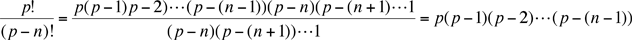Hi there,... I'm currently writing a time tabling algorithm for my phD. As part of the algorithm's rationale, I want to prove the following.... ASSUME n and p are natural numbers, and p >= n PROVE: pn>= (p!)/(p-n)! I proved what I think are the base cases (i.e. n = 0, and n = 1) but now I am stumped.... Any ideas anyone? Cheers Rhydian Rhydian,and hence you are asking if pn ≥ p(p-1)(p-2)···(p(n-1)) which it clearly is. Penny